##### Pre-Calculus Workbook For Dummies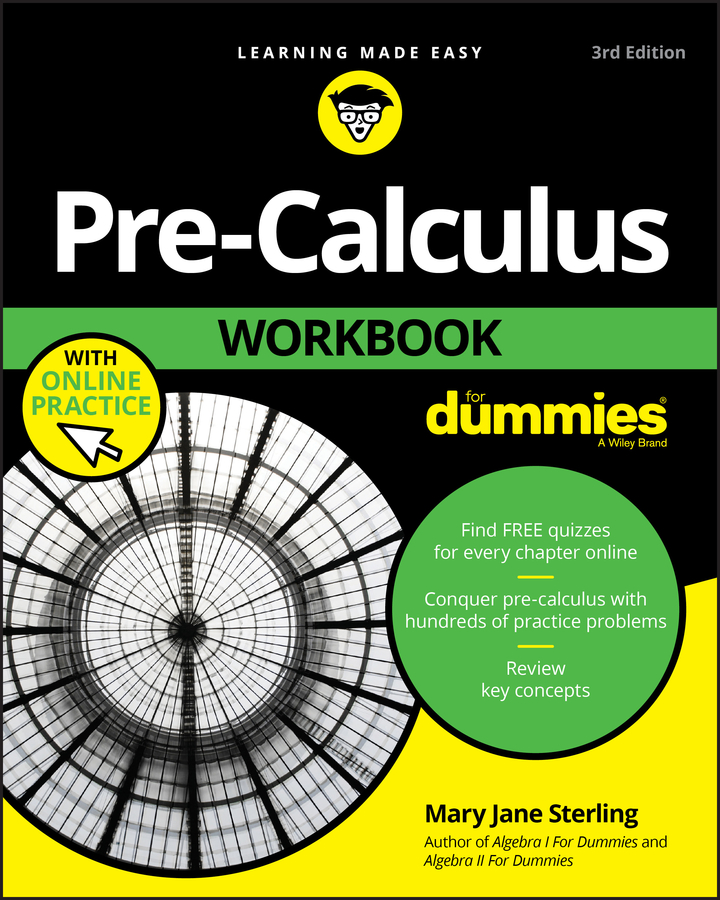You can incorporate reference angles into some other pre-calculus techniques to solve trig equations. One such technique is factoring. You've been factoring since algebra, so this process shouldn't be anything new. When confronted with an equation that's equal to 0 and a trig function that's being squared, or you have two different trig functions that are being multiplied together, you should try to use factoring to get your solution first. After factoring, you can use the zero-product property to set each factor equal to 0 and then solve them separately.

Try solving an example that involves factoring a trinomial 2 sin2 x + sin x – 1 = 0 using the following steps:

1. Let a variable equal the trig ratio and rewrite the equation to simplify.

Let u = sin x and rewrite the equation as 2u2 + u – 1 = 0.

2. Check to make sure that the equation factors.

Remember to always check for greatest common factor first.

The equation 2u2 + u – 1 = 0 factors to (u + 1)(2u – 1) = 0.

4. Switch the variables back to trig functions.

Rewriting your factored trig equation gives you (sin x + 1)(2 sin x – 1) = 0.

5. Use the zero-product property to solve.

If sin x + 1 = 0 then sin x = –1. If 2sin x – 1 = 0, then sin x = 1/2. Therefore,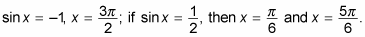In pre-calculus, you may be required to take the square root of both sides to solve a trigonometry function. For example, if you're given an equation such as 4 sin2 x – 3 = 0, follow these steps:

1. Let a variable equal the trig expression and rewrite the equation to simplify.

Let u = sin x and rewrite the equation as 4u2 – 3 = 0.

2. Isolate the trig expression.

For 4u2 – 3 = 0, add 3 to each side and divide by 4 on both sides to get u2 = 3/4.

3. Take the square root of both sides.

Don't forget to take the positive and negative square roots, which gives you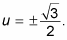4. Switch the variables back to trig functions.

Rewriting your factored trig equation gives you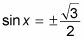5. Solve to find the reference angle.

The sine of x is both positive and negative for this example, which means that the solutions, or angles, are in all four quadrants. The positive solutions are in quadrants I and II, and the negative solutions are in quadrants III and IV. Use the reference angle in quadrant I to guide you to all four solutions.

If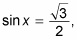the y value in the first quadrant is the long leg of the 30-60-90-degree triangle. Therefore, the reference angle is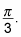6. Find the solutions.

Use the reference angle to find the four solutions: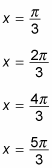Note that two of these solutions come from the positive value of sine and two come from the negative value of sine.